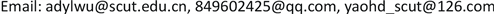ECLE-Commerce Letters2168-5843Scientific Research Publishing10.12677/ECL.2018.82008ECL-30100ECL20180200000_99226424.pdf经济与管理 一种基于社会化信任的改进的协同过滤推荐模型和方法 An Improved Collaborative Filtering Recommendation Model and Method Based on Social Trust 应良21开梅31怀栋41上海浦东发展银行海口分行，海南 海口华南理工大学经济与贸易学院电子商务系，广东 广州；华南理工大学现代服务业研究院商务智能研究中心，广东 广州华南理工大学经济与贸易学院电子商务系，广东 广州null0705201908026373© Copyright 2014 by authors and Scientific Research Publishing Inc. 2014This work is licensed under the Creative Commons Attribution International License (CC BY). http://creativecommons.org/licenses/by/4.0/

1华南理工大学经济与贸易学院电子商务系，广东 广州

2华南理工大学现代服务业研究院商务智能研究中心，广东 广州

3上海浦东发展银行海口分行，海南 海口Copyright © 2019 by author(s) and Hans Publishers Inc.

This work is licensed under the Creative Commons Attribution International License (CC BY).

http://creativecommons.org/licenses/by/4.0/1. 引言

2. 研究现状2.1. 国外研究现状

Massa等  首次提出了基于信任的推荐系统框架，他通过将项目评分矩阵和信任关系矩阵作为系统算法的输入项并提供给评分预测模型，最终得到了预测评分矩阵，改善了传统算法数据稀疏性问题，但其采用的是未加处理的布尔型信任标注值，表达信任的能力较弱，而且由此得到的信任关系也难以抵御恶意用户的攻击。Golbeck提出了一种TidialTrust算法，该算法通过广度优先搜索(BFS)的方法对信任传播方式进行了挖掘  。Massa等  在后来对TidialTrust进一步改进，提出了一种设定最大信任传递距离的MoleTrust算法。Zarghami等  提出了一种衡量信任程度的T指数，同时引入了信任传递机制，提高了推荐的精度和覆盖率。Jamali等  将基于项目的协同过滤推荐方法与信任推荐方法相结合，提出了TrustWalker随机游走算法，该算法认为信任程度较高的邻居用户比信任程度低的邻居用户在与源用户相似的目标项目上的评分更可靠，由此对源用户的未评分目标项目进行预测评分。Chen等  在传统协同过滤算法的基础上引入了信任的传播机制，提出了TPCF信任推荐模型。Bedi等  提出了一种信任动态更新的协同过滤推荐算法，通过用户的评分相似度和共同评分数占比综合推出。

2.2. 国内研究现状

3. 基于可信评分的矩阵预处理3.1. 可信评分计算

1) 个人可信度评分的计算

① 点评数可信度得分

PR _ CT ( u ) = m f ( u ) f s ( u ) × ( ( 1 − ε 1 ) + ε 1 × ∑ v = 1 v ≠ u k lg c t ( v ) | c t ( v ) − c t ( u ) | ) (1)

② 粉丝数可信度得分

PR _ FS ( u ) = m f ( u ) f s ( u ) × ( ( 1 − ε 2 ) + ε 2 × ∑ v = 1 v ≠ u k lg f s ( v ) | f s ( v ) − f s ( u ) | ) (2)

③ 关注数可信度得分

PR _ FW ( u ) = m f ( u ) f s ( u ) × ( ( 1 − ε 3 ) + ε 3 × ∑ v = 1 v ≠ u k lg f w ( v ) | f w ( v ) − f w ( u ) | ) (3)

④ 收藏数可信度得分

PR _ CN ( u ) = m f ( u ) f s ( u ) × ( ( 1 − ε 1 ) + ε 4 × ∑ v = 1 v ≠ u k lg c n ( v ) | c n ( v ) − c n ( u ) | ) (4)

⑤ 互粉数可信度得分

PR _ MF ( u ) = m f ( u ) f s ( u ) × ( ( 1 − ε 5 ) + ε 5 × ∑ v = 1 v ≠ u k lg m f ( v ) | m f ( v ) − m f ( u ) | ) (5)

ε 1 = c t ( v ) max [ c t ( n ) ] , ε 2 = f s ( v ) max [ f s ( n ) ] , ε 3 = f w ( v ) max [ f w ( n ) ] , ε 4 = c n ( v ) max [ c n ( n ) ] , ε 5 = m f ( v ) max [ m f ( n ) ] , n = 1 , 2 , ⋅ ⋅ ⋅ , k (6)

PR ( u ) = PR _ CT ( u ) + PR _ FS ( u ) + PR _ FW ( u ) + PR _ CN ( u ) + PR _ MF ( u ) (7)

2)互鉴可信度评分的计算

MI ( u ) = 1 − ρ + ρ × ∑ v = 1 v ≠ u k lg PR ( v ) | PR ( v ) − PR ( u ) | , u = 1 , 2 , ⋅ ⋅ ⋅ , k (8)

3.2. 社交关注矩阵可信量化

A social attention matrix based on mutual trus
ABCDE
ATA,B × MIBTA,C × MICTA,D × MIDTA,E × MIE
BTB,A × MIATB,C × MICTB,D × MIDTB,E × MIE
CTC,A × MIATC,B × MIBTC,D ×MIDTC,E × MIE
DTD,A × MIATD,B × MIBTD,C × MICTD,E × MIE
ETE,A × MIATE,B × MIBTE,C × MICTE,D × MID

3.3. 项目评分矩阵预填充

FR ( u , v ) = | UI u , v | | PI u , v | (9)

P u , i = r u ¯ + MI ( v ) × ( r v , i − r v ¯ ) (10)

r ′ u , i = r u ¯ + ∑ k ∈ R N u FR ( u , k ) × ( r k , i − r k ¯ ) ∑ k ∈ R N u FR ( u , k ) (11)

4. 基于可信评分预估矩阵的相似性计算4.1. 用户相似性计算

SIM _ A ( u , v ) = ∑ k ∈ PI u , v ( r u , k − r u ¯ ) ⋅ ( r v , k − r v ¯ ) ∑ k ∈ PI u , v ( r u , k − r u ¯ ) ⋅ ∑ k ∈ PI u , v ( r v , k − r v ¯ ) (12)

Sim _ A ( u , v ) = 1 + Sim _ A ( u , v ) 2 (13)

4.2. 预测评分计算

P u , i = r u ¯ + ∑ v ∈ NS ( u ) , RS ( i ) Sim _ A ( u , v ) × ( r v , i − r v ¯ ) ∑ v ∈ NS ( u ) , RS ( i ) Sim _ A ( u , v ) (14)

5. 算法实施步骤

STEP1-1可信评分的计算：根据推荐系统的用户数据库中，提取用户的点评数，关注数，粉丝数，收藏数，利用公式(1)至公式(7)离线计算每一位用户的五种影响因子得分和个人可信度评分，再利用公式(8)计算出每一位用户的互鉴可信度评分。

STEP1-2社交关注矩阵可信量化：将STEP1-1中计算出来的互鉴可信度融入到初始社交关注关系矩阵中，如表1所示，得到改善可信水平后的社会化信任矩阵。

STEP1-3项目评分矩阵预填充：将STEP1-1输出的互鉴可信度利用公式(9)至公式(11)，先转化为预估可信度后对用户未评分项目进行评分预测，并补充到初始用户项目评分矩阵的相应位置，最后得到数据密度改善的用户项目评分矩阵。

STEP2基于信任传播的相似性计算：基于STEP1-3填充后的项目评分矩阵，利用公式(12)至公式(13)计算相似度矩阵。

STEP3预测评分计算：根据公式(14)对用户的评分进行预测，将预测评分按照降序排序后形成推荐列表，位于前列的是目标用户最可能乐意接受的项目，以此进行实时的在线推荐。

6. 实验及结果分析6.1. 实验数据集和评估标准

Characteristic statistics of experimental data se

6.2. 实验结果讨论

1) 可信评分影响因子充分合理性验证

2) 社交关注和评分矩阵处理影响验证

Calculating MAE results with different influence factors of credible scor

PR_CTPR_FSPR_FWPR_CNPR_MF大众点评
0.792
0.751
0.778
0.776
0.791
0.743

MAE值取五个训练数据分组集计算的平均结果。测试分为三种情况：

① 未可信量化社交关注矩阵但预填充评分矩阵(No-Process-Trust)；

② 未预填充评分矩阵但可信量化社交关注矩阵(No-Filling-Rating)；

③ 既量化社交关注矩阵又预填充评分矩阵(Process-Trust-Rating)。

7. 结论及展望